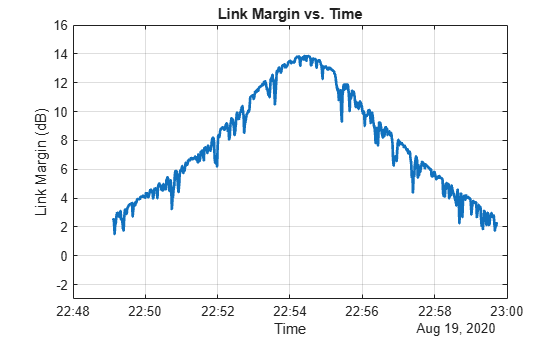# ebno

Eb/No at final node of link

## Syntax

``e = ebno(lnk)``
``e = ebno(lnk,timeIn)``
``[e,timeOut] = ebno(___)``

## Description

example

````e = ebno(lnk)` returns a matrix `e` of the history of received values for energy per bit to noise power spectral density (Eb/No) in dB at the final node in each possible multihop link in the vector `lnk`. The rows of the matrix correspond to the link object in `lnk` and the columns correspond to the time sample.```
````e = ebno(lnk,timeIn)` returns a column vector of Eb/No `e` in dB at the final node in each link defined in the vector `lnk` at the specified datetime `timein`. Each element of `e` corresponds to a link in `lnk`. If no time zone is specified in `timeIn`, the time zone is assumed to be Coordinated Universal Time(UTC).```

example

````[e,timeOut] = ebno(___)` returns the received Eb/No values and the corresponding times in UTC.```

## Examples

collapse all

Create a satellite scenario object.

```startTime = datetime(2021,12,12,10,42,0); stopTime = startTime + days(1); sampleTime = 5000; % seconds sc = satelliteScenario(startTime,stopTime,sampleTime);```

Add a satellite to the scenario.

```semiMajorAxis = 10000000; % meters eccentricity = 0; inclination = 0; % degrees rightAscensionOfAscendingNode = 0; % degrees argumentOfPeriapsis = 0; % degrees trueAnomaly = 210; % degrees sat = satellite(sc,semiMajorAxis,eccentricity, ... inclination,rightAscensionOfAscendingNode, ... argumentOfPeriapsis,trueAnomaly);```

Add a transmitter to the satellite.

`tx = transmitter(sat);`

Add a ground station to the scenario.

```latitude = 0; % degrees longitude = 30; % degrees gs = groundStation(sc,latitude,longitude);```

Add a receiver to the ground station.

`rx = receiver(gs,MountingAngles=[0; 180; 0]);`

`lnk = link(tx,rx);`

Get the Eb/No history at the receiver and the time samples.

`[e,t] = ebno(lnk)`
```e = 1×19 -Inf -Inf -Inf -Inf -Inf -Inf -Inf -Inf -Inf -Inf -Inf -Inf -Inf -Inf -Inf -Inf -Inf -Inf -Inf ```
```t = 1x19 datetime Columns 1 through 3 12-Dec-2021 10:42:00 12-Dec-2021 12:05:20 12-Dec-2021 13:28:40 Columns 4 through 6 12-Dec-2021 14:52:00 12-Dec-2021 16:15:20 12-Dec-2021 17:38:40 Columns 7 through 9 12-Dec-2021 19:02:00 12-Dec-2021 20:25:20 12-Dec-2021 21:48:40 Columns 10 through 12 12-Dec-2021 23:12:00 13-Dec-2021 00:35:20 13-Dec-2021 01:58:40 Columns 13 through 15 13-Dec-2021 03:22:00 13-Dec-2021 04:45:20 13-Dec-2021 06:08:40 Columns 16 through 18 13-Dec-2021 07:32:00 13-Dec-2021 08:55:20 13-Dec-2021 10:18:40 Column 19 13-Dec-2021 10:42:00 ```

This example demonstrates a simple technique for incorporating random pointing loss at the transmit and receive antennas in a space-to-ground radio link. Caluclate the link margin without pointing loss, and then you add the random sequences of pointing loss to the result.

Define parameters for pointing error simulation.

```freq = 8; % Carrier frequency, GHz satAntDiam = 0.4; % Satellite antenna diameter, m satAntEff = 0.43; % Satellite antenna efficiency, (0,1] satAntPtErr = 0.5; % Satellite antenna std. dev. pointing error, deg gsAntDiam = 2.7; % Ground antenna diameter, m gsAntEff = 0.63; % Ground antenna efficiency, (0,1] gsAntPtErr = 0.2; % Ground antenna std. dev. pointing error, deg gsNoiseTemp = 150; % Ground system noise temperature, Kelvin reqEbNo = 13.0; % Required Eb/No for 8PSK BER=1e-5, decibels```

Create a satellite scenario object.

```startTime = datetime(2020,8,19,22,35,0); stopTime = datetime(2020,8,19,23,15,0); sampleTime = 1; % Seconds sc = satelliteScenario(startTime,stopTime,sampleTime);```

Add satellite orbital elements for a sun-synchronous satellite.

```sat = satellite(sc,7*1e6,0.0002178,97.8356,359.1795,135.7406,224.3991); satTx = transmitter(sat,Frequency=freq*1e9,BitRate=60,Power=3); satAnt = gaussianAntenna(satTx,DishDiameter=satAntDiam, ... ApertureEfficiency=satAntEff); gs = groundStation(sc,Latitude=69.649208,Longitude=18.955324, ... Altitude=0.35,MinElevationAngle=5.0); gsGim = gimbal(gs,MountingAngles=[0;180;0], ... MountingLocation=[0;0;-5]); waveLen = physconst("lightspeed")/(freq*1e9); pkG = 10*log10(gsAntEff*((pi*gsAntDiam)/waveLen)^2); % Peak antenna gain, dBi gsRx = receiver(gsGim,MountingLocation=[0;0;1], ... GainToNoiseTemperatureRatio=pkG-10*log10(gsNoiseTemp), ... RequiredEbNo=reqEbNo); pointAt(sat,gs); pointAt(gsGim,sat); lnk = link(satTx,gsRx); [e, time] = ebno(lnk); ```

Generate a random sequence of pointing losses for transmit and receiver antennas.

```satAntPtErrRad = deg2rad(satAntPtErr); satRanAngle = (satAntPtErrRad)*randn(1,length(time)); % Random pointing angle error, radians satRanAngle = filter(ones(1,10)/sqrt(10),1,satRanAngle); % Model control loop filtering satBeamWidth = (waveLen/(satAntDiam*sqrt(satAntEff))); % 3 dB beamwidth, radians satPeakGain = 10*log10(satAntEff*((pi*satAntDiam)/waveLen)^2); % Peak gain, dBi satRanAntGain = 10*log10(satAntEff*(((pi.*satAntDiam)./ ... waveLen).^2).*exp(-2.76*((satRanAngle./satBeamWidth).^2))); % Antenna gain at off-boresight angle, dBi, % from Satellite Communications, Gagliardi satRanPtLoss = satPeakGain - satRanAntGain; % Random pointing loss, dB gsAntPtErrRad = deg2rad(gsAntPtErr); gsRanAngle = (gsAntPtErrRad)*randn(1,length(time)); % Random pointing angle error, radians gsRanAngle = filter(ones(1,10)/sqrt(10),1,gsRanAngle); % Model control loop filtering gsBeamWidth = waveLen/(gsAntDiam*sqrt(gsAntEff)); % 3 dB beamwidth, radians gsPeakGain = 10*log10(gsAntEff*((pi*gsAntDiam)/waveLen)^2); % Peak gain, dBi gsRanAntGain = 10*log10(gsAntEff*(((pi.*gsAntDiam)./ ... waveLen).^2).*exp(-2.76*((gsRanAngle./gsBeamWidth).^2))); % Antenna gain at off-boresight angle, dBi, % from Satellite Communications, Gagliardi gsRanPtLoss = gsPeakGain - gsRanAntGain; % Random pointing loss, dB ```

Compute composite pointing losses. ` `

`totRanPtLoss = satRanPtLoss + gsRanPtLoss; `

Compute the link margin.

```margin = e-totRanPtLoss-gsRx.RequiredEbNo; plot(time,margin,LineWidth=2); title("Link Margin vs. Time"); ylim([-3 16]); xlabel("Time"); ylabel("Link Margin (dB)"); grid on;```## Input Arguments

collapse all

Link analysis object, specified as a `Link` object vector or scalar.

Time at which the output is calculated, specified as a `datetime` scalar. If no time zone is specified in `timeIn`, the time zone is assumed to be UTC.

## Output Arguments

collapse all

Energy per bit to noise power spectral density (Eb/No), returned as a scalar, vector, or matrix. If `timeIn` is not specified, `e` is a row vector or a matrix.

Time samples of the output Eb/No, returned as a scalar or vector. If time history of Eb/No is returned, `timeOut` is a row vector.

Note

When the `AutoSimulate` property of the satellite scenario is `true`, the Eb/No history from `StartTime` to `StopTime` is returned. When the property is `false`, the Eb/No history from `StartTime` to `SimulationTime` is returned.

## Algorithms

The Eb/No in dB is calculated as

EbNo = txPower + txAntennaGain - txSystemLoss - pathloss + rxAntGaintoNoiseTempRatio - 10*log10(K) - rxSystemLoss - 10*log10(bitRate) - 60

where:

• txPower is the transmitter power in dBW.

• txAntennaGain is the transmitter antenna gain in dB.

• txSystemLoss is the transmitter system loss in dB.

• pathloss is the path loss in dB.

• rxAntGaintoNoiseTempRatio is the receiver antenna gain to noise temperature ratio.

• K is the Boltzmann constant.

• rxSystemLoss is the receiver system loss in dB.

• bitRate is the bit rate in Mbps.

## Version History

Introduced in R2021a